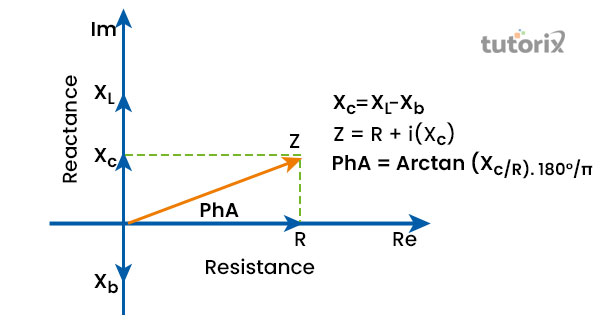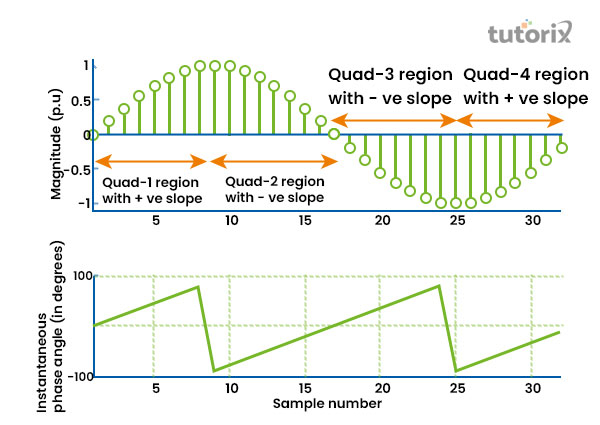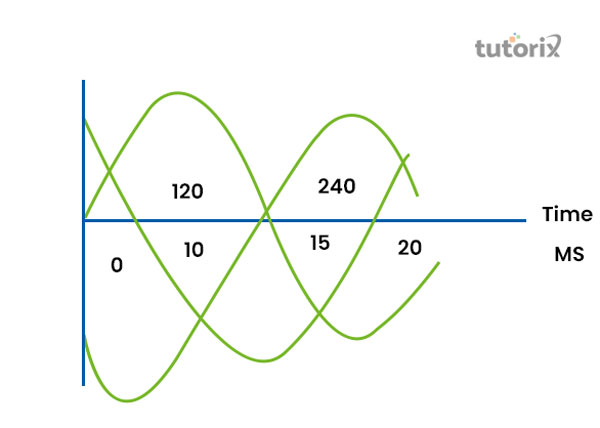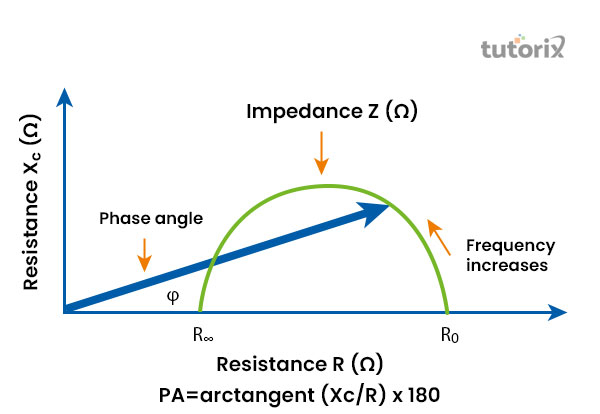# Phase Angle

## Introduction

Phase angle represents a particular point in the time cycle that measures the zero arbitrary to the expression as an angle.

Periodic wave is the characteristic of the phase angle. The displacement of the periodic wave is connected with the process of transformation is connected with the periodic variation and with the time.

It is also measured with angular units such as degrees or radians. The implementation of signals in the phase angle helps identify an appropriate filter, a dc voltage is delivered to the Phase Angle analogously. In this tutorial, differences between phases and the way of measurement are available.Figure 1: Nature of Phase Angle

## Information Regarding Phase Angle

A phase angle has the characteristics of a periodic wave. It is equivalent to the phrase in numerous belongings. The angular component of the periodic wave is understood as the Phase Angle. The displacement in the periodic wave is characterised as the variation in the periodic with the relation to time or distances, in some cases, it can be both. When the periodic wave repeats continuously, it helps identify its different attributes, such as frequency, period, and amplitude.

A phase difference is referred to as the difference between the frequencies of two waves within the same point of time (Electrical4u, 2022). The angular component of the periodic wave is referred to as the phase angle also.

"A∠θ, where A is referred to as the magnitude and θ symbolises the wave of phase angle". The "Instantaneous Phase Angle" (IPA) is computed by utilising the arctan ranges from −90° to +90°. IPAs in one rotational cycle that is nearly zero during the steady-state procedure. Additionally, during a transient situation, both current signals and voltage are misinterpreted, and consequently, their "Derived Phase Mean Index" (DPMIs) organise useful info regarding the transient.Figure 2: Samples of IPA and its signal

## Measurement of a Phase Angle

Different dimensions of electric voltages are the primary reasons to measure the proportions of the voltage triangle with the phase angle. The timing uncertainty between two periodic stimulations is estimated. The phase distinction between two identical frequencies and constituents without a dc can easily interpret. The process of measurement is followed by different metrics in order to analyse the factors including the percentage calculation (Allaboutcircuits, 2022).

Measuring the material uncertainty is estimated among "two periodic signals". With the fraction of measurement, the amount of unit in angular is about 360 degrees or a full cycle.

## Fundamental of calculating Phase Angle

From the component of fundamentally each waveform, the estimation of Phase Angle is possible, both of the signals in the distortion can consequently lead to mistakes. Calculating these angles may express as complicated, however, it is straightforward with accurate instructions. The frequency guides the times the wave will depart via a given establishment in a limited time (Sciencing, 2022).

Depending on the method, the magnitude direction can lead to the misshaping of both or either signal and result errors. The magnitude is depending on the distortion with the method of measuring. In the time of calculating the phase difference with the angular measurement, it is vital to note the wave and "reference point". It can help in representing another wave or waveform.

## Phase Difference

The time interval which represents one wave is beforehand from another waveform while calculating the phase angle, it is defined as the phase difference.

• A complete phase is referred to as the 360 degrees or it can be "2π radians" and it represents by the letter 'ɸ' (Electrical4u, 2022).
• For instance, if two waves have the same frequency of time in a three-phase circuit, they are described as Phase R and Phase B, both phases have a 50 Hz frequency.

Let's assume, phase R start from 0 deg and phase B starts from 120 deg. Hence, the difference phase will be 0 deg - 120 deg or - 120 deg. In this case, R will be considered the "reference phase".Figure 3: Phase difference

The followings are the two vital terms in forming the concept of phase waves.

The difference between the two waves is in the phase quadrature only when the difference between the phases is about 90 deg, and it can be negative or positive.

### "Phase Opposition"

The difference between the phases is regarded with the same frequency and it can be either negative or positive 180 deg. In this situation, the phases will be placed opposite each other.

### "Bioelectric impedance"

The analysis of humans studied the bioelectrical impedance for a calculator process in the phase angle to make changes in the resistance power. The calculated phase angle is depending on different phases of biological factors.Figure 4: Bioelectric impedance

## Connections of "Resonance Circuit"

The followings are the circuit with connection to phase descending on the current and voltage that belongs to the RLC circuit with the below-furnished relations.

### Resistor

The voltage and current are resistors in the same phase. Consequently, the phase distinctions between these portions attending in a resistor construct the value as zero.

### Capacitor

The voltage and current in the same phase that represents inside a capacitor do not rely on each other in the same phase. Current in the tools can lead to the voltage by 90 deg.

### Inductor

In the inductor, the voltage and current respect each other and cannot be situated in the same phase. In this phase, the voltage in a device is 90 deg ahead of the current (Sciencedirect, 2022). Hence, the phase difference with the context of current and voltage the inductor results as 90 deg.

## Conclusion

In this tutorial, the importance of the phase angle has been discussed with the importance of phase wave in forming different types of measurement. In the practical use case of science, the presentation of the phase angle represents the accurate value for a waveform based on the fraction of the period. Forming different waveforms by calculating the phases can be easy with the application of the phase angle formula. In the projection of the vector, the real axis which is used in a diagram to calculate phase angle is referred to as the "reference point".

Q1. What is phase angle in physics?

The specific point which is represented in the time cycle for measuring the difference in time is called the phase angle. It help recognises the difference between current and voltage.

Q2. What is phase difference?

The intermission of one wave from another waveform is estimated to calculate the difference in physics is defined as the phase difference. The phase difference is the property of the "multiple waveforms".

Q3. How to measure phase angle measure?

The phase angle in physic is used to measure the significant difference between the voltage and electricity with the help of degrees or radians. The utilisation of the "reference point" is mandatory in this measurement.

Q4. What is phase shift?

The small variation among the forms of a wave is considered the "phase shift". The existence of delay between two waveforms is "Phase Shift".

## References

### Journals

Kumar, Bandi & Mohapatra, Abheejeet & Chakrabarti, Saikat & Kumar, Avinash. (2021). Phase angle-based fault detection and classification for protection of transmission lines. International Journal of Electrical Power & Energy Systems. 10.1016/j.ijepes.2021.107258. Retrieved from: https://www.researchgate.net

Websites